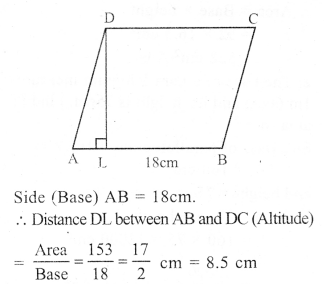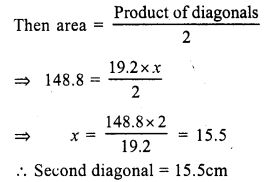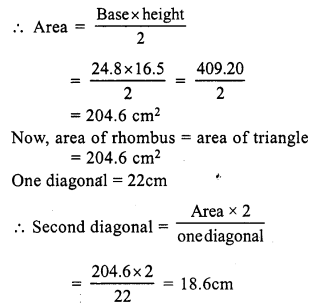# RS Aggarwal Class 7 Solutions Chapter 20 Mensuration Ex 20C

In this chapter, we provide RS Aggarwal Solutions for Class 7 Chapter 20 Mensuration Ex 20C for English medium students, Which will very helpful for every student in their exams. Students can download the latest RS Aggarwal Solutions for Class 7 Chapter 20 Mensuration Ex 20C Maths pdf, free RS Aggarwal Solutions Class 7 Chapter 20 Mensuration Ex 20C Maths book pdf download. Now you will get step by step solution to each question.

### RS Aggarwal Solutions for Class 7 Chapter 20 Mensuration Ex 20C Download PDF

Question 1.
Solution:
In parallelogram ABCD,
Base AB = 32cm
Height DL = 16.5cm.Area = Base x height = 32 x 16.5 cm² = 528 cm²

Question 2.
Solution:
Base of parallelogram = 1 m 60m = 160 cm
and height = 75 cm
Area = Base x height = 160 x 75 = 12000 cm²
= 1200010000 m² = 1.2m²

Question 3.
Solution:
Base of parallelogram = 14dm = 140cm
and height = 6.5 dm = 65cm
Area (in cm²) = Base x height = 140 x 65 = 9100 cm²
Area (in m²) = 140100 x 65100 = 910010000
= 0.91 m²

Question 4.
Solution:
Area of parallelogram = 54 cm²
Base = 15 cmQuestion 5.
Solution:
Area of parallelogram ABCD = 153 cm²Question 6.
Solution:
In parallelogram ABCD
AB || DC and AD || BC and AB = DC, AD = BC
AB = DC = 18cm, BC = 12cm
Area of parallelogram ABCD = Base x altitude = DC x AL = 18 x 6.4cm2 = 115.2 cm²
and area of parallelogram ABCD = BC x AM
⇒ 115.2 = 12 x AM
⇒ AM = 9.6 cm

Question 7.
Solution:
In parallelogram ABCD
AB = DC = 15 cm
BC = AD = 8 cm.Distance between longer sides AB and DC is 4cm
i.e. perpendicular DL = 4cm.
DM ⊥ BC.
Area of parallelogram = Base x altitude = AB x DL = 15 x 4 = 60 cm²
Again let DM = x cm
area ABCD = BC x DM = 8 x x = 8x cm²
8x cm² = 60 cm²
⇒ x = 7.5 cm
Distance between shorter lines = 7.5 cm

Question 8.
Solution:
Let Base of the parallelogram = x⇒ x² = 108 x 3 = 324 = (18)²
⇒ x = 18
Base = 18 cm
and altitude = 13 x 18 = 6 cm

Question 9.
Solution:
Area of parallelogram = 512 cm²
Let height of the parallelogram = x
Then base = 2x
Area = Base x height
⇒ 512 = 2x x x
⇒ 2x² = 512
⇒ x² = 256 = (16)²
⇒ x = 16
Base = 2x = 2 x 16 = 32 cm
and height = x = 16 cm

Question 10.
Solution:
(i) Each side of rhombus = 12 cm
height = 7.5 cmArea = Base x height = 12 x 7.5 = 90 cm²
(ii) Each side = 2 cm = 20 cm
Height = 12.6 cm
Area = Base x height = 20 x 12.6 = 252 cm²

Question 11.
Solution:
(i) Diagonals of rhombus ABCD are 16 cm and 28 cmQuestion 12.
Solution:
In rhombus ABCD, diagonals AC and BD intersect each other at right angles at O.
AO = OC and BO = ODAO = 12 x AC = 12 x 24 cm = 12cm
Let OB = x
Each side of rhombus = 20cm
In right ∆AOB
AO² + OB² = AB² (Pythagoras Theorem)
⇒ (12)² + OB² = (20)²
⇒ 144 + OB² = 400
⇒ OB² = 400 – 144 = 256 = (16)²
⇒ OB = 16
But BD = 2 BO = 2 x 16 = 32cm
Now, area of rhombus = Productofdiagonals2
= 24×322 cm2 = 384 cm²

Question 13.
Solution:
Area of rhombus = 148.8 cm²
one diagonal = 19.2 cm
Let second diagonal = xQuestion 14.
Solution:
Area of rhombus = 119 cm²
Perimeter = 56 cm
Its side = 564 = 14 cmQuestion 15.
Solution:
Area of rhombus = 441 cm²
Height = 17.5 cmQuestion 16.
Solution:
Base of a triangle = 24.8 cm
Corresponding height = 16.5 cmAll Chapter RS Aggarwal Solutions For Class 7 Maths

—————————————————————————–

All Subject NCERT Exemplar Problems Solutions For Class 7

All Subject NCERT Solutions For Class 7

*************************************************

I think you got complete solutions for this chapter. If You have any queries regarding this chapter, please comment on the below section our subject teacher will answer you. We tried our best to give complete solutions so you got good marks in your exam.

If these solutions have helped you, you can also share rsaggarwalsolutions.in to your friends.